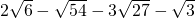Adding and subtracting radicals is similar to adding and subtracting variables. The condition is that the variables, like the radicals, must be identical before they can be added or subtracted. Recall the addition and subtraction of like variables:

Example 9.3.1

Simplify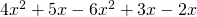.

First, we sort out like variables and reorder them to be combined.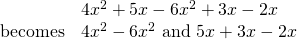Combining like variables yields: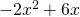When adding and subtracting radicals, follow the same logic. Radicals must be the same before they can be combined.

Example 9.3.2

Simplify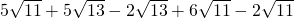.

First, we sort out like variables and reorder them to be combined.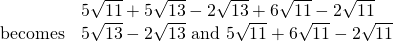Combining like radicals yields: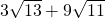Generally, it is required to simplify radicals before combining them. For example:

Example 9.3.3

Simplify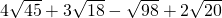.

All of these radicals need to be simplified before they can be combined.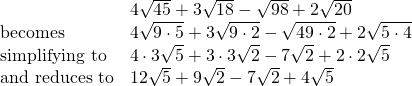Recombining these so they can be added and subtracted yields: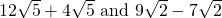Combining like radicals yields: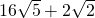Higher order radicals are treated in the same fashion as square roots. For example:

Example 9.3.4

Simplify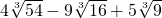.

Like example 9.3.3, these radicals need to be simplified before they can be combined.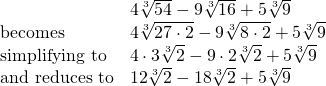Combining like radicals yields: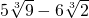# Questions

Simplify.

1.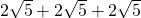2.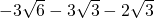3.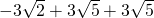4.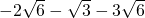5.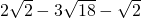6.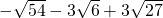7.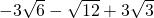8.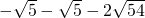9.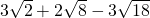10.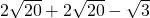11.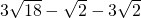12.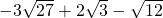13.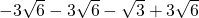14.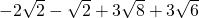15.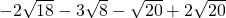16.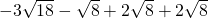17.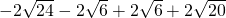18.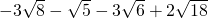19.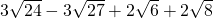20.